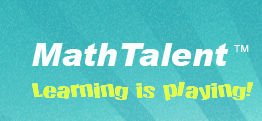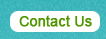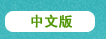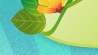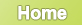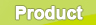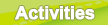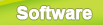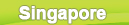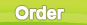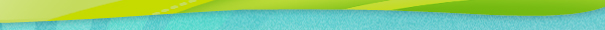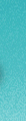Product

### 1. What's MathTalentTM?

The MathTalent method is a revolutionary way for children to learn math. MathTalent has helped hundreds of thousands of children worldwide progress rapidly in arithmetic and mathematics. The MathTalent method is based on children's visual memorization capacity through games with specially-designed cards. The suite includes the deck of cards, a workbook, and a CD:

• 54 cards. The workbook contains illustrations that help students understand the meanings of the numbers, mathematical concepts and techniques to solve arithmetic problems. With hundreds of exercises, it can serve as a reference book as well as a workbook. It covers addition, subtraction, multiplication, division. The forty group activities can be played among a group of children at school or between parents and children at home.

• A companion workbook. Containing hundreds of exercises, the workbook has exercises and answers for every challenge children will encounter.

• A software package. The software includes 86 games that currently only run on PC's; a MAC version is under development. Each game may have up to 9 options for teachers or parents to select, so that the computer-based games can be played equally at home or in school. As the child moves a card on top of the appropriate space holder, the screen displays the matching arithmetic operation; in addition to a standard score, the game keeps track of the time elapsed and the number of wrong moves, thus enabling parents and teachers to objectively judge the progress of the student. The CD also includes a complete HELP file.

### 2. What results can be achieved using MathTalent?

The effectiveness of the MathTalent method is truly remarkable: we boast that children as young as 4 years old will master addition and subtraction after playing with MathTalent for just 24 hours, and that they can master multiplication and division after another 12 hours. Our claim is backed by a money - back guarantee, but we have never received a request for refund. On the contrary, parents have praised MathTalent after their kids mastered the four basic arithmetic operations in 6 months - a feat by any child education standard.

### 3. Is teaching MathTalent hard?

MathTalent requires minimal training: a professional teacher will easily learn the basics in a two-hour session.

### 4. What's the MathTalent's philosophy?

MathTalent is truly revolutionary in its approach to introducing children to arithmetic. MathTalent is based on visualization and association: between the ages of 4 and 8, children are actually faster than adults at recognizing colors and basic shapes, but they lack abstract logical thinking. The MathTalent figures and games were developed based on children's intuitive visual and memorization capabilities, as a vehicle for introducing the basic concepts of number hierarchy, addition, subtraction, and commutation.

For example, the card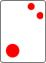has one big red circle and two smaller ones, three red circles in all. It illustrates that 3 is a combination of 1 and 2. Teachers guide students to count the big circle first, then the two small circles to show that 1 + 2 = 3, or count the small circles first then the big one to show that 2 + 1 = 3. Covering the big circle illustrates that 3 - 1 = 2 or covering the small circles illustrates that 3 - 2 = 1. Students grasp the concepts of addition/subtraction and master the meanings of the numbers by referring to the cards, images that easily remain in their long-term memory.

Even as they develop an intuitive understanding of a hierarchic order amongst numbers, children learn arrangements between cards that introduce the two basic operations, adding and subtracting. The figures on the cards naturally represent different possible arrangements and combinations for each number, which leads kids to recognize each number as an entity that can be split in different ways. Thereafter, the specially-designed exercises build on this foundation to easily introduce more advanced arithmetic concepts, including carrying, borrowing, multiplying, and dividing.

In summary, MathTalent works with children's keen visual senses to help them develop an intuitive organizational framework, which is then associated with arithmetic functions and the hierarchy of numbers. The group activities are designed to enable teachers to easily split the class into different levels and allocate more time to those groups that require more coaching. All the while, the exercises are also designed to empower children to discuss and coach one another within their own group.

### 5. What are the differences between MathTalent and other mathematical learning methods?

MathTalent is a completely different mathematical learning method than traditional ways.

• MathTalent is 2-dimensional. A picture is worth a thousand words and it's very true for children learning math.

At age 4 - 8 children can recognize colors and basic shapes but lack abstract logical thinking. Teaching them math should be based on what they know, not what they don't. So how do children feel when they count 1, 2, 3 ...10? They have no idea what they are talking about. Still can't put yourself in children's shoes? OK, let's do an experiment. Think of a number, how about 5? Yes you see in your mind that 5 is 1 + 4, or 2 + 3, or 6 - 1, or two 5s are 10 etc. You know all the possibilities. Now let's do a mapping, use a letter to represent a number, i.e. a is 1, b is 2, c is 3, d is 4, e is 5, ... and j is 10 . Then let me ask you, what's e? Can you still see that e is a + d, or b + c, or f - a? This is exactly how children feel. How many times has your child told you 3 plus 5 is 8 and you were very happy, but the next day he/she forgot completely? And how?many times have you seen your child add 2 numbers by counting fingers? The key problem is that the traditional way teaches children to learn math literally. When children learn 1 + 2 = 3, they are remembering a phrase 1 plus 2 equals 3, and they don't understand the mathematical meanings involved. What are mathematical meanings involved? 1 + 2 = 3 implies that 2 + 1 = 3, 3 - 1 = 2 and 3 - 2 = 1 and children should understand these all together. This shows how traditional math learning method is not effective or, as a matter of fact, is wrong. The traditional way is 1-dimensional or logical, which students are not good at or don't even carry the ability at their young ages.

MathTalent bridges the gap through the specially designed flash cards. Each card has been carefully designed for and used by tens of thousands children to reflect students?intellectual characteristics at age 4 to 8, thus a number is no longer an abstract symbol but a realistic graphic. This helps children understand the mathematical concepts and children won't just memorize the results literally. Children learn math from what they know.

• MathTalent is playing.

Interest is the best motivation for Children to learn. Learning should be a pleasant experience not a frustrating one. When children encounter difficulties they get frustrated and lose interest. They won't learn anything if they are not enjoying it. If they are left behind, they will never catch up. So give them a jump-start before they go to school.

Children won't get frustrated playing MathTalent flash cards since they are not dealing with abstract numbers. At an early stage they may count the shapes on the cards, that's fine. Gradually, actually very quickly, they can recognize the 50 cards without counting. Believe it or not children have better graphic-recognizing ability than adults. This is theoretically correct and our experiments have shown this to be true. Once children can recognize the cards they are 50% done learning calculation. How hard is that?

What's the best time for children to learn? When they fall sleep? Absolutely not. Yes, the best time to learn is when they are excited and concentrated. Nothing can better intrigue children than activities and games. They learn to compare numbers through activities, they learn addition and subtraction through activities, they learn multiplication and division through activities, and they will learn arithmetic through activities. In the meantime they learn to compete with each other, which would greatly improve their calculating speed and have themselves well trained for school. They also learn quick math tricks and other social life and science knowledge while solving computation problems on the workbook, like the fact that tadpoles grow up to become frogs. Happy learning is the key.

• MathTalent is precise.

Why do children make mistakes when they do a calculation? Why are they slow? The answer to the first question is they don't know how to do it, the answer to the second question is they need practice. The MathTalent's approach involves 2 aspects:

• Do it right.

If children don't understand how to do it, they will make mistakes. For example, to do 7 + 9 right, he/she needs to know that 7 is 1 and 6, add 1 to 9 to get 10, then add 6 to 10 is 16. Sound complicated? Not in MathTalent. To split 7 into 1 and 6 when adding 7 to 9 is called Red Method, one of the 4 methods used in MathTalent. How do they know 7 is 6 and 1? They will know it once they can recognize the Red 7 card. MathTalent does not teach children addition or subtraction first, but starts off with illustrating the component parts of 1 to 10 via the flash cards. Unlike the normal way that teaches 1 + 6 = 7, children will know 7 can have different pairs of components parts, i.e. 7 is 1 and 6 (represented by Red Card 7), 7 is 2 and 5 (represented by Blue Card 7), and 7 is 3 and 4 (represented by Green Card 7). So how do they know 1 + 9 is 10? They will know it once they can recognize Red 10 card. Now 9 + 7 = 9 + 1 + 6 = 10 + 6 = 16 is an obvious answer.

If children keep making the same type of errors, MathTalent has answers to that type of errors. For instance, if a child always fails to add 8 to another number, like 8 + 7, the?Green Method and Green 7 card should be reviewed. Traditionally we would ask he/she keep practicing, this does not work well here since he/she may know 8 + 7 is 15 but still make mistakes when do 18 + 27 later. Children need to understand how to do it right, not just memorize.

• Do it fast.

First of all, is it important? Yes, you bet. Arithmetic and numbers are the building blocks of all math and science courses. Feeling comfortable with these basic concepts will give your children an edge in any future class. Recall how many points we have lost during exams due to wrong computations or not having enough time to finish all the questions?

Doing more exercises normally won't help. As a matter of fact children should not waste time on that. What matters is the response, the response of finding the component parts of a number and using the correct method to solve the problem. Let's try 15 + 8 in MathTalent way.

The children's first response is to use the Blue Method to add 5 to 8, their second response is to correctly split 5 into 2 and 3 according to Blue Method, add 2 to 8 to get 10 and 3 in the ones. Their last response is to add the 10 from 15 to the 10 from 2 + 8, and get the final answer is 23.

Their first response is easy to get since there are only 4 methods in MathTalent when adding 2 numbers with a sum greater than 10. They're based on the bigger number of the 2 addends. Fro details see page 23 of the workbook.

Their second response is the key, and this is the strength of MathTalent. Whenever children see a card they will be impressed with the structure of a number. For example every time children look at Green 10 card they can tell there are 2 parts of the card, one part has 2 bigger shapes and the other has 8 smaller shapes. They will learn this is a 10, a 10 consists of 2 and 8.

Their third response can be gained through activities and exercises on the workbook. We have illustrations of how to carry the 1 from adding the ones to add the tens.

Sound complicated? Not at all. Can you imagine a 5-year-old kid can add up the 50 cards and get the right answer within?45 seconds? I have to admit I may not pull it off even though I have a master's degree in math, but more than 80% of children can accomplish it. Most likely you won't accomplish it either, but I am pretty sure your child can do it.

• MathTalent is detailed.

Children will encounter various challenges while learning math. When they make mistakes like 15 + 7 = 26 or 13 + 18 = 21, what do we do? Just tell them the answers and let them practice again? That's the typical way but not the good way, children may just remember it for now and forget it again. If you ask them to do it again they may still get it wrong, most likely they don't understand it at all. Let's try the MathTalent way.

First of all we need to know these are two different type of mistakes, thus need to be treated differently. The first one, 15 + 7 = 26, tells that children can't add 5 to 7 correctly, but do know that the sum (5+7) is more than 10 and carry the 10 to add to the 10 from 15. Now let's focus on 5 + 7. We have the following steps:

• Learn Blue 5 card and Blue 10 card. The Blue 5 card will teach children that 5 is 2 and 3. Page 13 of the workbook has illustrations for Blue 5 card. The Blue 10 card will teach that 10 is 3 and 7. Page 21 of the workbook has the illustrations for Blue 10 card.

• Learn the Blue Method to split 5 into 3 and 2, add 3 to 7 to get 10 and carry over, and the ones is 2. Page 23 of the workbook has illustrations and page 26 has exercises for the Blue Method.

The second one, 13 + 18 = 21 tells that children can add the ones right but fail to carry the 10 from the adding of the ones. Page 30 of the workbook p30 has the illustrations of adding double-digit numbers.

In general MathTalent answers to every mistake children may make in learning arithmetic. With MathTalent children understand the concepts and grasp the correct method to do math, but not waste time on tedious repeating math exercises.

### 4. Contact

MathTalent Corp
33 Lee Street
Cambridge, MA 02139, USA
Tel: (857)244-1027